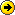"This translation is 75% up-to-date"

# Measuring translation progress in the CLWE Project

It is useful for the users of a wiki translation system to be able to know how much a given translation is up-to-date with respect to other-language versions of the same page which may feature modifications that have not propagated.

In full generality, more than one page may be a source of changes. Let us first consider the case where there are only two languages for a given page. There are many different ways to compute a "translation metric" between two pages. Given a source version S and a target version T, let us agree that the translation metric between them is zero when T is empty, and one (or 100%) when nothing in S needs translation into T.

# Computing a translation metric in the CLWE architecture

Huberdeau's translation syncing architecture (Architecture Description (PDF)) provides two sources of input that could feed into a translation metric. The first is the set of content elements that have been introduced elsewhere in the translation set, but have not yet been propagated to the translation under consideration. Since the content elements have labels but not explicit representations in this architecture, the most that can be derived from them is a number of changes that need to be propagated.

The second source of input is the content diff that is computed to enable an update between two translations. The diff is comprised of the changes between two versions of S that require propagation into T. It is represented as text segments that were removed or added. Content diffs have the advantage of providing strings of characters whose length give an approximate measure of required effort. Obviously, an empty diff would correspond to a metric of 100%.

Mathematically, let us use diff+(S,T) to represent the total number of characters that appear in additions in the diff and diff-(S,T) represent the total number of characters whose removal needs to propagate into T.

One possible translation metric is

M(S,T) = Len(T) / ( Len(T) + diff+(S,T) + diff-(S,T)/N ) (1)

where N is a fudge factor representing the ratio of effort between translating a chunk of text into and removing it from a translation (Does N=10 seem reasonable?).

A picture is worth a thousand words. Imagine that the state in which the target page finds itself after it is updated is represented as a rectangle that has two segments glued together.

```===================================+++++++++++++++++++
^^ current version of T ^^       ^ diff+ & diff- ^```

The first segment represents the current size of T, and the second represents the changes that need to be propagated. The ratio of Len(T) to the total length is our translation metric.

We hypothesize that in most cases it will give an accurate assessment of the work required. A few shortcomings are obvious, though. For instance, if T is long but most of the required update work consists in completely replacing T by something short, the metric will be too high (optimistic). Also, the computed content diff occasionally includes changes that are already present in the target and should not be counted; these would artificially lower the metric.

## Examples of applying the above metric with N=10

 Source(old) Source(current) Target Metric aaa aaab (+1) aaa 3/(3+1)=75% aaa aa (-1) aaa 3/(3+1/10)=97% aaa aaab (+1) 0/(0+1)=0% aaa aaabb (+2) cccccccc 8/(8+2)=80% aaa a (-2) cccccccc 8/(8+2/10)= 98%

Applying the translation metric on real pages

## Working with multiple languages

Changes to a given target page may be required arising from updates at source pages in more than one language. We can combine the two-language metrics to obtain an assessment of the total work required to bring the target up to date with respect to all other translations.

Because of the possibility of overlap, the changes coming from the different language pages fall on a spectrum ranging from completely independent (all the changes occur only in one language) to very dependent (all the changes are redundant once those in one of the pages have been propagated). This can be complicated to assess very precisely.

(Work-in-progress) Here are three possible formulas, with N the number of translations in the translation set.

M(T) = product(M(Si,T)) for all Si in the translation set (2)

M(T) = min ( Sum(M(Si,T)) - N + 1 , 0) (3)

M(T) = min ( M(Si,T) ) over all i (4)

Formulas 2 and 3 should work better in the case where independent changes need to be integrated. Formula 3 corresponds to adding together the translation deficits (1-M(Si,T)).

Formula 4 assumes complete redundancy of the changes, and amounts to saying that translation would be complete if initiated from the source page that has the most changes pending propagation.

# Computing and caching

• Reuse the procedure for finding the appropriate version to display the content diff
• Compute upon saves and store, to avoid unnecessary computation.Home PageDemo screencastLast changesCLWE To doForum Wiki-translationBabelWiki WorkshopArchitecture document (pdf)CLWE Paper (pdf)